Updating search results...

# 19 Results

View
Selected filters:
• MCCRS.Math.Content.6.NS.B.4
Remix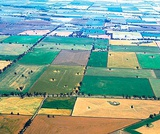Conditional Remix & Share Permitted
CC BY-NC
Rating
0.0 stars

Students use a geometric model to investigate common factors and the greatest common factor of two numbers.Key ConceptsA geometric model can be used to investigate common factors. When congruent squares fit exactly along the edge of a rectangular grid, the side length of the square is a factor of the side length of the rectangular grid. The greatest common factor (GCF) is the largest square that fits exactly along both the length and the width of the rectangular grid. For example, given a 6-centimeter × 8-centimeter rectangular grid, four 2-centimeter squares will fit exactly along the length without any gaps or overlaps. So, 2 is a factor of 8. Three 2-centimeter squares will fit exactly along the width, so 2 is a factor of 6. Since the 2-centimeter square is the largest square that will fit along both the length and the width exactly, 2 is the greatest common factor of 6 and 8. Common factors are all of the factors that are shared by two or more numbers.The greatest common factor is the greatest number that is a factor shared by two or more numbers.Goals and Learning ObjectivesUse a geometric model to understand greatest common factor.Find the greatest common factor of two whole numbers equal to or less than 100.

Subject:
Numbers and Operations
Material Type:
Lesson Plan
Author:
Angela Vanderbloom
07/05/2018
RemixConditional Remix & Share Permitted
CC BY-NC
Rating
0.0 stars

Lesson OverviewStudents use a geometric model to investigate common multiples and the least common multiple of two numbers.Key ConceptsA geometric model can be used to investigate common multiples. When congruent rectangular cards with whole-number lengths are arranged to form a square, the length of the square is a common multiple of the side lengths of the cards. The least common multiple is the smallest square that can be formed with those cards.For example, using six 4 × 6 rectangles, a 12 × 12 square can be formed. So, 12 is a common multiple of both 4 and 6. Since the 12 × 12 square is the smallest square that can be formed, 12 is the least common multiple of 4 and 6.Common multiples are multiples that are shared by two or more numbers. The least common multiple (LCM) is the smallest multiple shared by two or more numbers.Goals and Learning ObjectivesUse a geometric model to understand least common multiples.Find the least common multiple of two whole numbers equal to or less than 12.

Subject:
Numbers and Operations
Material Type:
Lesson Plan
Author:
Angela Vanderbloom
07/07/2018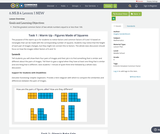Conditional Remix & Share Permitted
CC BY-NC
Rating
0.0 stars

Goals and Learning ObjectivesFind the greatest common factor of two whole numbers equal to or less than 100.

Subject:
Mathematics
Material Type:
Lesson Plan
Author:
Angela Vanderbloom
08/22/2018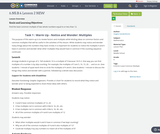Conditional Remix & Share Permitted
CC BY-NC
Rating
0.0 stars

Goals and Learning ObjectivesFind the least common multiple of two whole numbers equal to or less than 12.

Subject:
Mathematics
Material Type:
Lesson Plan
Author:
Angela Vanderbloom
08/22/2018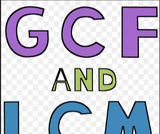Conditional Remix & Share Permitted
CC BY-NC
Rating
0.0 stars

In this lesson, students apply what they have learned about factors and multiples to solve a variety of problems. In the first activity, students to use what they have learned about common factors and common multiples to solve less structured problems in context (MP1).

Subject:
Mathematics
Material Type:
Lesson Plan
Author:
Angela Vanderbloom
07/09/2018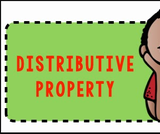Conditional Remix & Share Permitted
CC BY-NC
Rating
0.0 stars

Students will learn to use the distributive property to rewrite each sum as a product.  Visual representations of the areas of rectangles and their respective measurements (length and width) will be used.

Subject:
Mathematics
Material Type:
Lesson Plan
Author:
Angela Vanderbloom
07/10/2018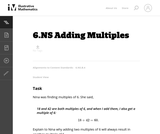Unrestricted Use
CC BY
Rating
0.0 stars

Subject:
Mathematics
Material Type:
Activity/Lab
Provider:
Illustrative Mathematics
Provider Set:
Illustrative Mathematics
Author:
Illustrative Mathematics
05/01/2012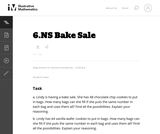Unrestricted Use
CC BY
Rating
3.0 stars

This series of word problems requires students to apply the concepts of factors and common factors in a context.

Subject:
Mathematics
Material Type:
Activity/Lab
Provider:
Illustrative Mathematics
Provider Set:
Illustrative Mathematics
Author:
Illustrative Mathematics
05/01/2012
Remix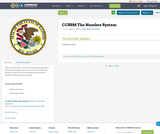Conditional Remix & Share Permitted
CC BY-SA
Rating
0.0 stars

This resource links to both the Fractions progression document published by the Common Core Writing Teams in June 2011 and the Module posted on the Illustrative Mathematics website.

Material Type:
Assessment
Homework/Assignment
Teaching/Learning Strategy
Author:
ISBE Math Specialists
08/08/2019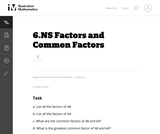Unrestricted Use
CC BY
Rating
0.0 stars

This problem uses the same numbers and asks essentially the same mathematical questions as "6.NS Bake Sale," but that task requires students to apply the concepts of factors and common factors in a context.

Subject:
Mathematics
Material Type:
Activity/Lab
Provider:
Illustrative Mathematics
Provider Set:
Illustrative Mathematics
Author:
Illustrative Mathematics
05/01/2012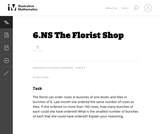Unrestricted Use
CC BY
Rating
0.0 stars

This task provides a context for some of the questions asked in "6.NS Multiples and Common Multiples." A scaffolded version of this task could be adapted into a teaching task that could help motivate the need for the concept of a common multiple.

Subject:
Mathematics
Material Type:
Activity/Lab
Provider:
Illustrative Mathematics
Provider Set:
Illustrative Mathematics
Author:
Illustrative Mathematics
05/01/2012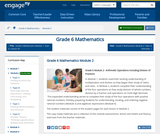Conditional Remix & Share Permitted
CC BY-NC-SA
Rating
0.0 stars

In Module 1, students used their existing understanding of multiplication and division as they began their study of ratios and rates.  In Module 2, students complete their understanding of the four operations as they study division of whole numbers, division by a fraction and operations on multi-digit decimals.  This expanded understanding serves to complete their study of the four operations with positive rational numbers, thereby preparing students for understanding, locating, and ordering negative rational numbers (Module 3) and algebraic expressions (Module 4).

Subject:
Numbers and Operations
Material Type:
Module
Provider:
New York State Education Department
Provider Set:
EngageNY
09/21/2013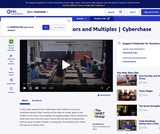Educational Use
Rating
0.0 stars

Harry and his students look at various arrangements of classroom desks in this video from Cyberchase.

Subject:
Mathematics
Material Type:
Lecture
Provider:
PBS LearningMedia
Provider Set:
PBS Learning Media: Multimedia Resources for the Classroom and Professional Development
Author:
U.S. Department of Education
WNET
07/09/2008Conditional Remix & Share Permitted
CC BY-NC
Rating
0.0 stars

Subject:
Mathematics
Material Type:
Full Course
Provider:
Pearson
10/06/2016Rating
0.0 stars

Expressions

Type of Unit: Concept

Prior Knowledge

Students should be able to:

Write and evaluate simple expressions that record calculations with numbers.
Use parentheses, brackets, or braces in numerical expressions and evaluate expressions with these symbols.
Interpret numerical expressions without evaluating them.

Lesson Flow

Students learn to write and evaluate numerical expressions involving the four basic arithmetic operations and whole-number exponents. In specific contexts, they create and interpret numerical expressions and evaluate them. Then students move on to algebraic expressions, in which letters stand for numbers. In specific contexts, students simplify algebraic expressions and evaluate them for given values of the variables. Students learn about and use the vocabulary of algebraic expressions. Then they identify equivalent expressions and apply properties of operations, such as the distributive property, to generate equivalent expressions. Finally, students use geometric models to explore greatest common factors and least common multiples.

Subject:
Mathematics
Algebra
Material Type:
Unit of Study
Provider:
PearsonConditional Remix & Share Permitted
CC BY-NC
Rating
0.0 stars

Lesson OverviewStudents use a geometric model to investigate common multiples and the least common multiple of two numbers.Key ConceptsA geometric model can be used to investigate common multiples. When congruent rectangular cards with whole-number lengths are arranged to form a square, the length of the square is a common multiple of the side lengths of the cards. The least common multiple is the smallest square that can be formed with those cards.For example, using six 4 × 6 rectangles, a 12 × 12 square can be formed. So, 12 is a common multiple of both 4 and 6. Since the 12 × 12 square is the smallest square that can be formed, 12 is the least common multiple of 4 and 6.Common multiples are multiples that are shared by two or more numbers. The least common multiple (LCM) is the smallest multiple shared by two or more numbers.Goals and Learning ObjectivesUse a geometric model to understand least common multiples.Find the least common multiple of two whole numbers equal to or less than 12.

Subject:
Numbers and Operations
Material Type:
Lesson Plan
Provider:
Pearson
09/21/2015Conditional Remix & Share Permitted
CC BY-NC
Rating
0.0 stars

Students use a geometric model to investigate common factors and the greatest common factor of two numbers.Key ConceptsA geometric model can be used to investigate common factors. When congruent squares fit exactly along the edge of a rectangular grid, the side length of the square is a factor of the side length of the rectangular grid. The greatest common factor (GCF) is the largest square that fits exactly along both the length and the width of the rectangular grid. For example, given a 6-centimeter × 8-centimeter rectangular grid, four 2-centimeter squares will fit exactly along the length without any gaps or overlaps. So, 2 is a factor of 8. Three 2-centimeter squares will fit exactly along the width, so 2 is a factor of 6. Since the 2-centimeter square is the largest square that will fit along both the length and the width exactly, 2 is the greatest common factor of 6 and 8. Common factors are all of the factors that are shared by two or more numbers.The greatest common factor is the greatest number that is a factor shared by two or more numbers.Goals and Learning ObjectivesUse a geometric model to understand greatest common factor.Find the greatest common factor of two whole numbers equal to or less than 100.

Subject:
Numbers and Operations
Material Type:
Lesson Plan
Provider:
Pearson
09/21/2015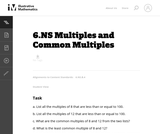Unrestricted Use
CC BY
Rating
0.0 stars

This problem uses the same numbers and asks similar mathematical questions as "6.NS The Florist Shop," but that task requires students to apply the concepts of multiples and common multiples in a context.

Subject:
Mathematics
Material Type:
Activity/Lab
Provider:
Illustrative Mathematics
Provider Set:
Illustrative Mathematics
Author:
Illustrative Mathematics
05/01/2012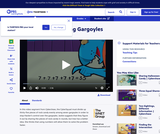Educational Use
Rating
3.0 stars

The CyberSquad must divide 35 candies evenly among seven gargoyles in this video segment from Cyberchase.

Subject:
Mathematics
Material Type:
Lecture
Provider:
PBS LearningMedia
Provider Set:
PBS Learning Media: Multimedia Resources for the Classroom and Professional Development
Author:
U.S. Department of Education
WNET